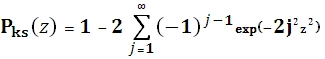# SQL Server Kolmogorov-Smirnov cumulative distribution function

KSP

Updated: 29 June 2012

Use KSP to calculate the Kolmogorov-Smirnov (KS) cumulative distribution function. The equation for the cumulative distribution function is:Syntax
SELECT [wctStatistics].[wct].[KSP] (
<@z, float,>)
Arguments
@z
is the value at which to evaluate the function. @z is an expression of type float or of a type that can be implicitly converted to float
Return Types
Float
Remarks
·         @z must greater than or equal to 0
·         Pks(0) = 0
·         Pks(8) = 1
Examples
SELECT wct.KSP(1) as KSP
This produces the following result.
KSP
----------------------
0.730000328322645

### SupportCopyright 2008-2023 Westclintech LLC         Privacy Policy        Terms of Service